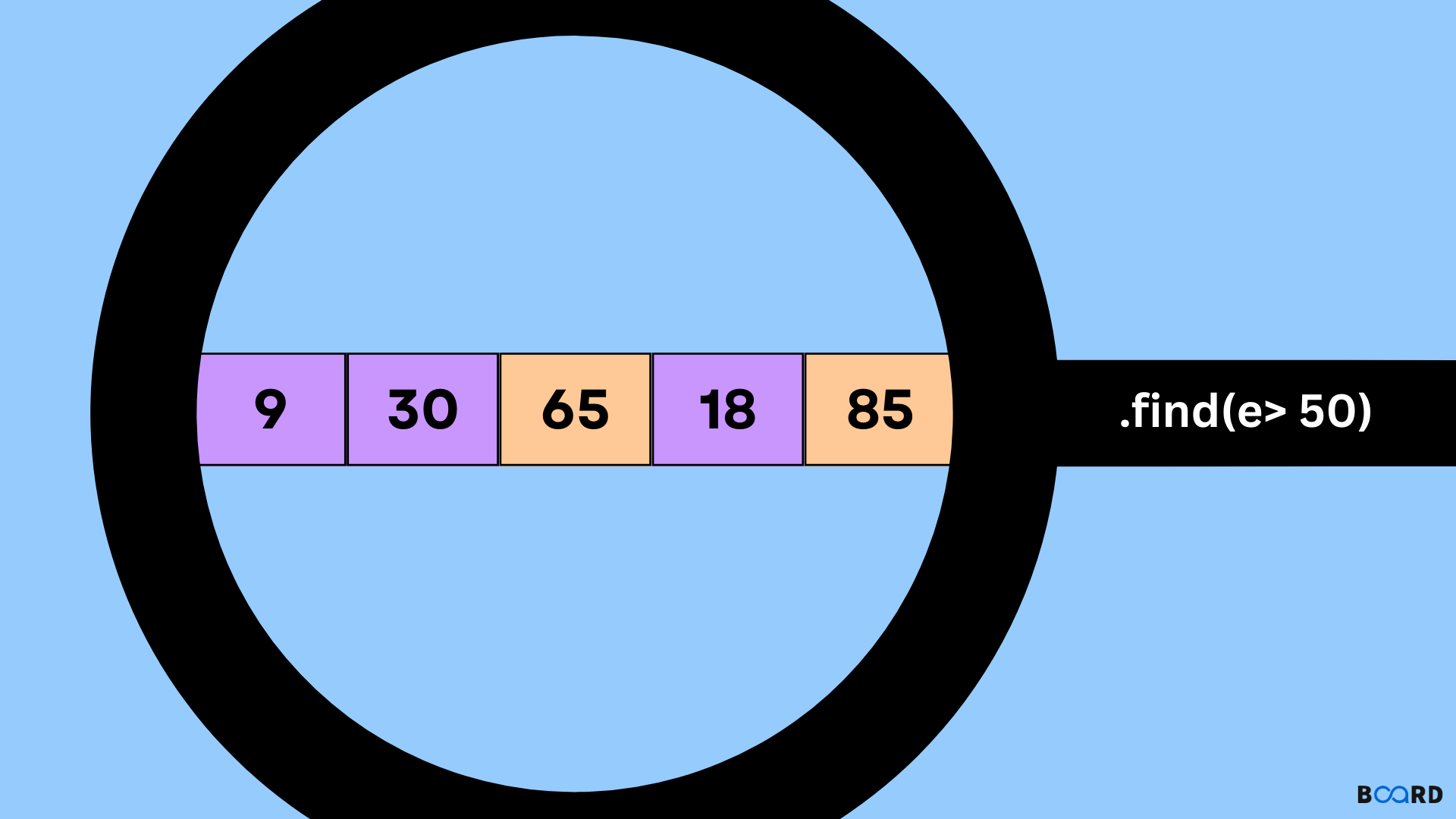# Array find() method in JavaScript## Introduction

The find() method of an array JavaScript object finds the first element in the array that satisfies a specified test and returns that element. If the test returns true, then the return value is null; otherwise, it is the value of its associated index position. The find() method is a very useful function that we can use to search for items in an array.We can use the find() method to search for values in an array. It will return the first item it finds, or null if nothing is found.

The find() method returns the first element in the provided array that satisfies the provided testing function. If no values satisfy the testing function, undefined is returned.If no argument is passed, it will return the first element in the array that matches your criteria (if there are multiple matching elements). If an argument is passed, it will return the first element in the array that matches your criteria (if there are multiple matching elements).

The syntax is:

 array.find(function(currentValue, index, arr),thisValue);

## Parameters

function : (Required)A function to run for each array element.

currentValue :(Required) This parameter holds the value of current element.

index: (optional)holds the index of the current element.

arr : (optional)holds the array object the current element belongs to.

thisValue: (optional)Default undefined.A value passed to the function as its this value.

where index is an integer or a negative number, and value can be any type of expression including strings and booleans.

## Examples

Example 1:

 var array = [-55, -20, 7, -90, -78];     var n = array.find(function (element) {        return element > 0;    });     console.log(n);

Output:

 7
write your code here: Coding Playground

Example 2:

 const array1 = [50, 12, 80, 130, 4];const n= array1.find(element => element > 20);console.log(n);

Output:

 50
write your code here: Coding Playground

Example 3:

 function Prime(element, index, array) {  let n = 2;  while (n <= Math.sqrt(element)) {    if (element % n++ < 1) {      return false;    }  }  return element > 1;}console.log([40, 16, 4, 8].find(Prime)); console.log([6, 3, 20, 12].find(Prime));

Output:

 3
write your code here: Coding Playground

## Important Points

• If you need the index of the found element in the array, use findIndex().
• If you need to find the index of a value, use indexOf(). (It's similar to findIndex(), but checks each element for equality with the value instead of using a testing function.)
• If you need to find if a value exists in an array, use includes(). Again, it checks each element for equality with the value instead of using a testing function.
• If you need to find if any element satisfies the provided testing function, use some().

## Conclusion

The find() method of an array is used to retrieve a specific value from the array. The find() method takes two arguments: a value and an index. The index argument is the position of the value in the array; the value argument is the value you want to return. If your code returns false, then it means that there are no items with the specified values at that index.